161106 Epaper

 A Section B SectionC Section D Section161105 Epaper

 A Section B SectionC Section D Section161104 Epaper

 A Section B SectionC Section D Section161103 Epaper

 A Section B Section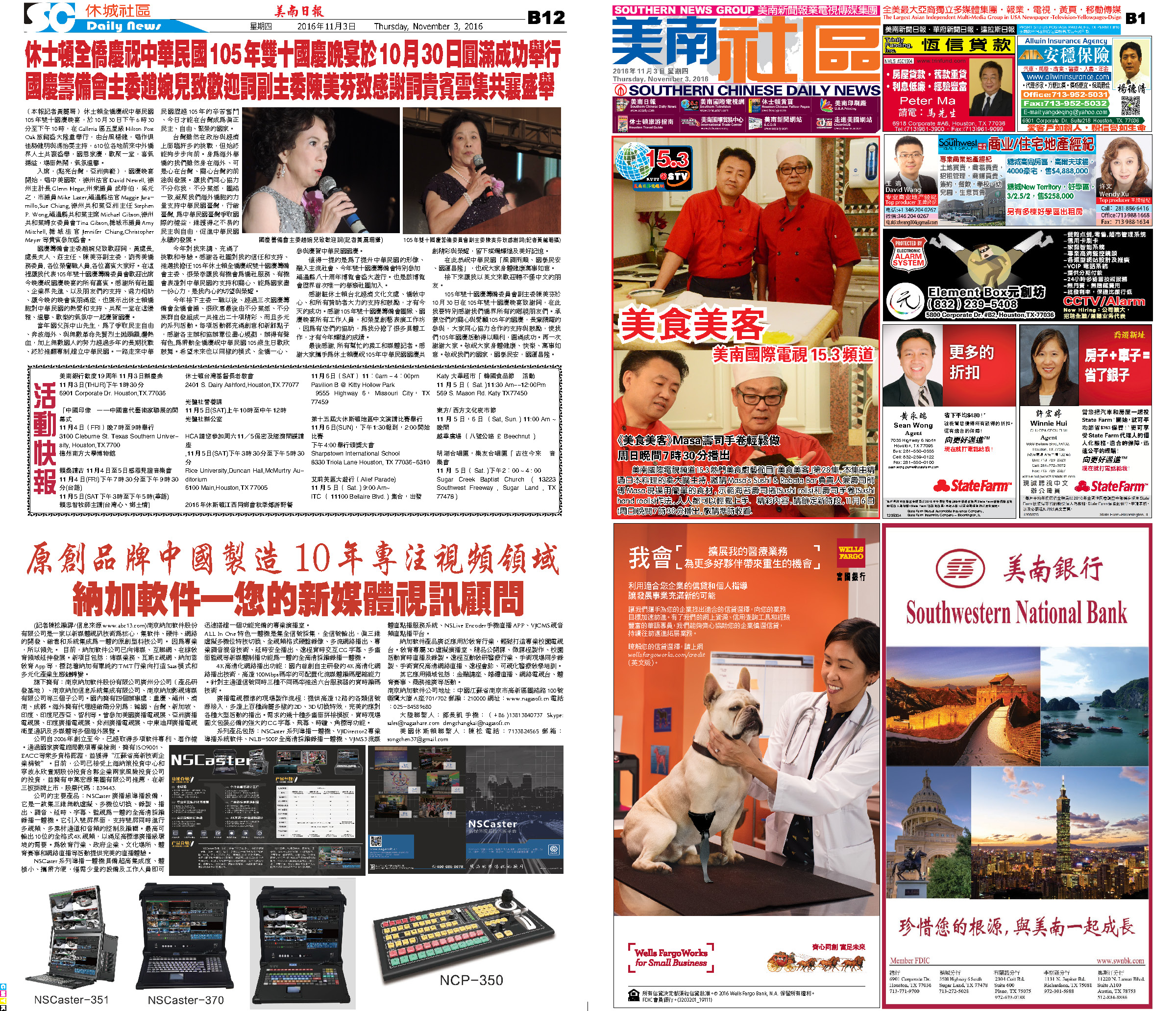C Section D Section161102 Epaper

 A Section B SectionC Section D Section161101 Epaper

 A Section B SectionC Section D Section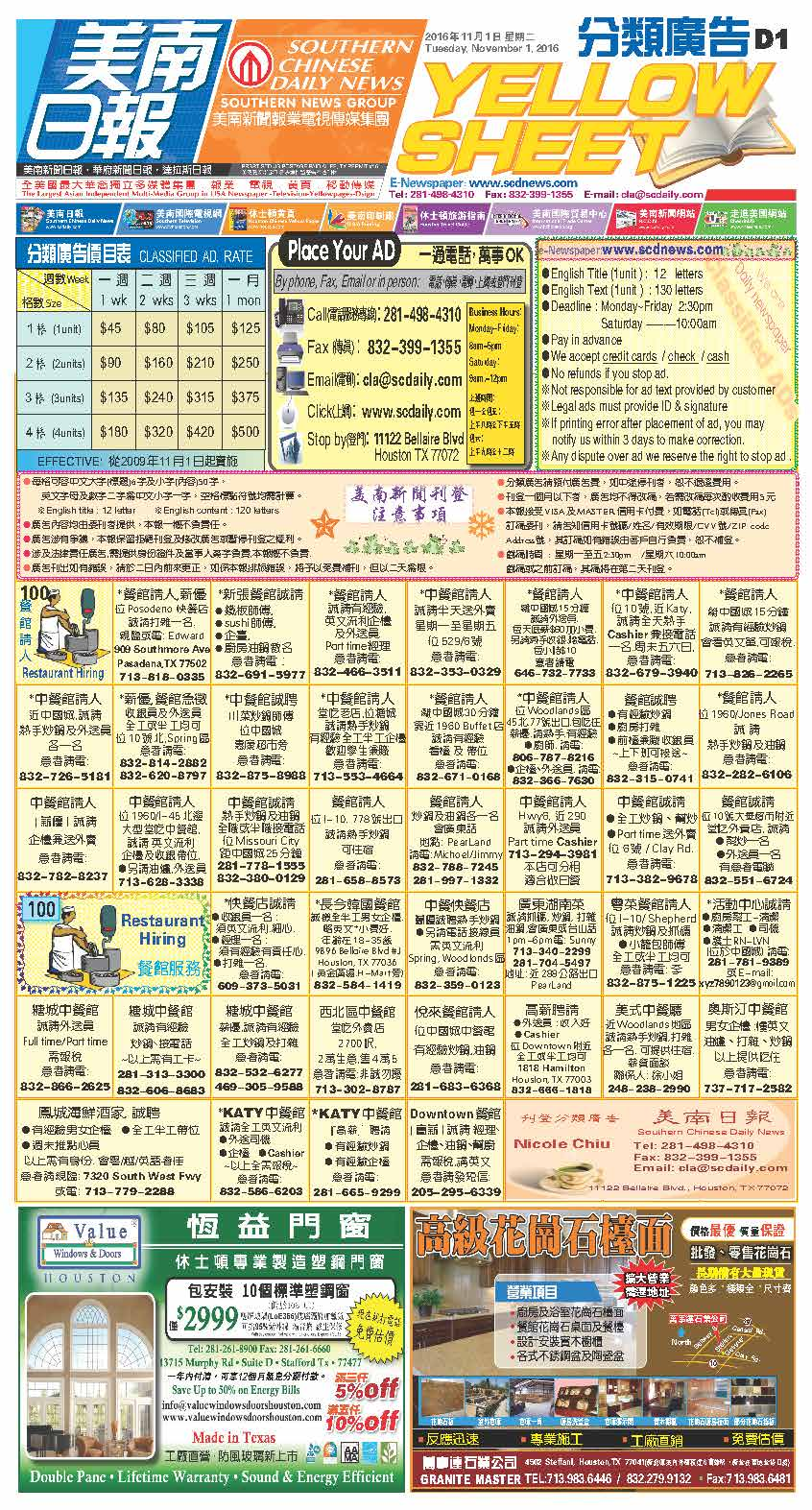161031 Epaper

 A Section B SectionC Section D Section161030 Epaper

 A Section B SectionC Section D Section161028 Epaper

 A Section B SectionC Section D Section161029 Epaper

 A Section B SectionC Section D Section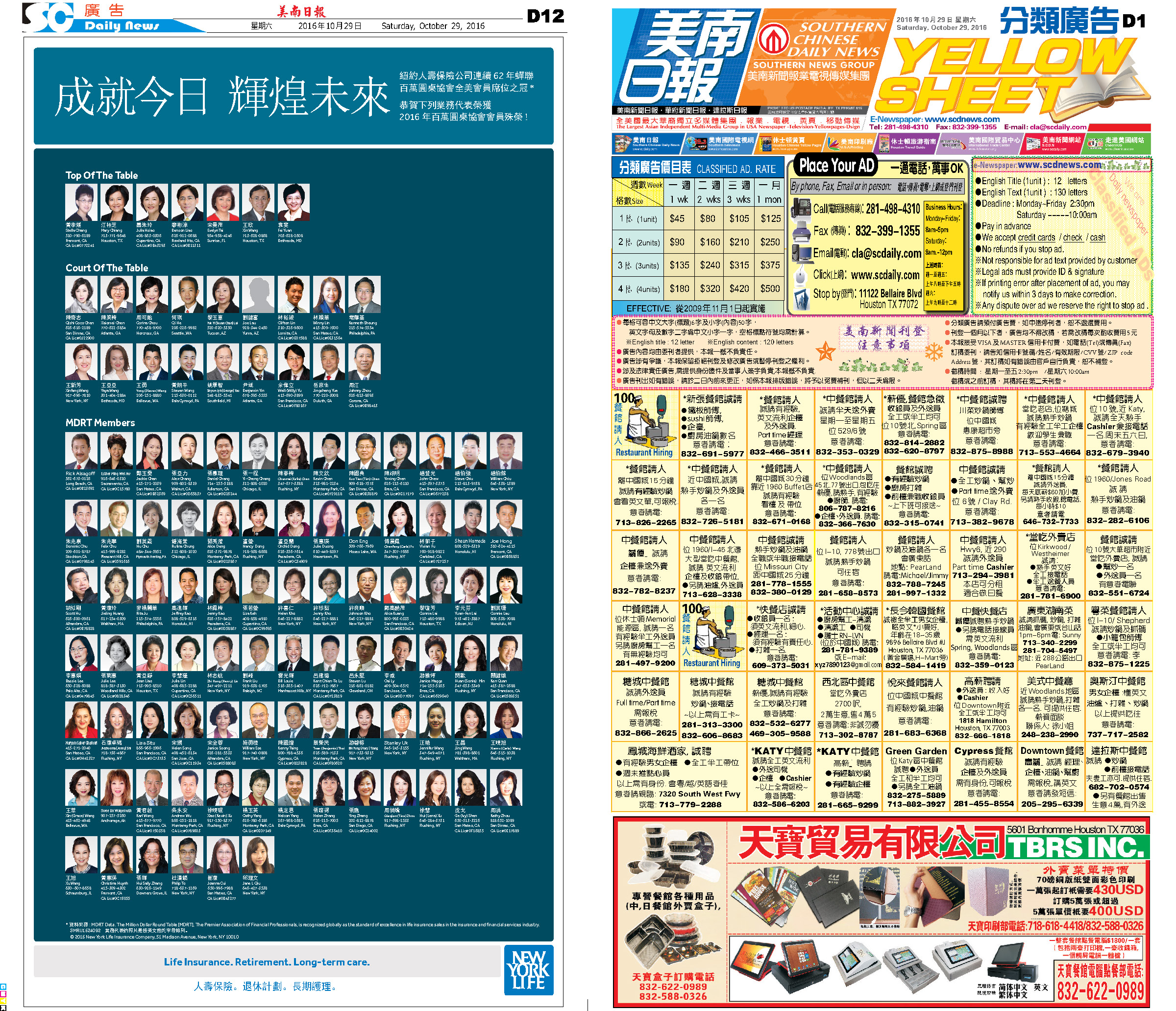161027 Epaper

 A Section B Section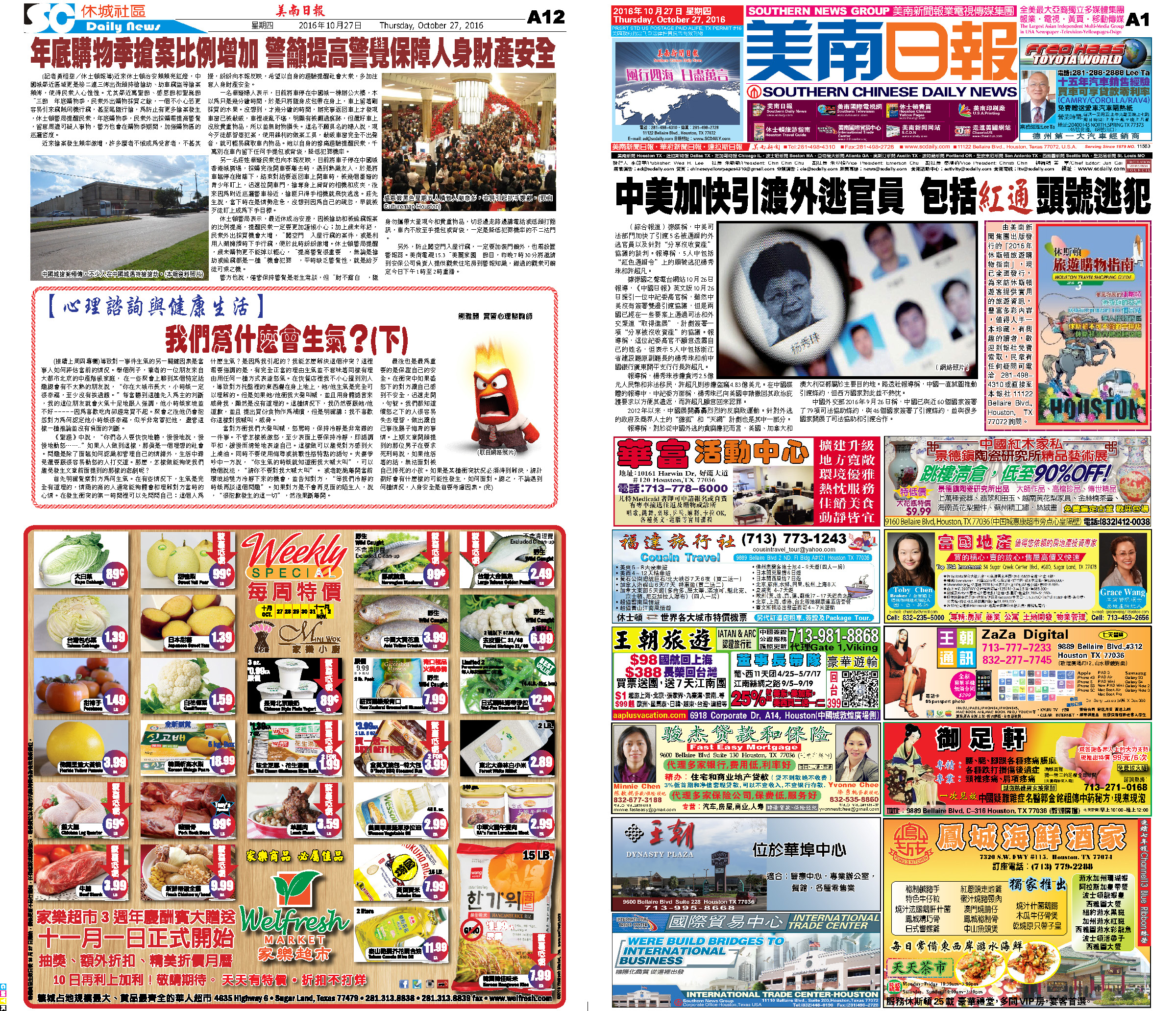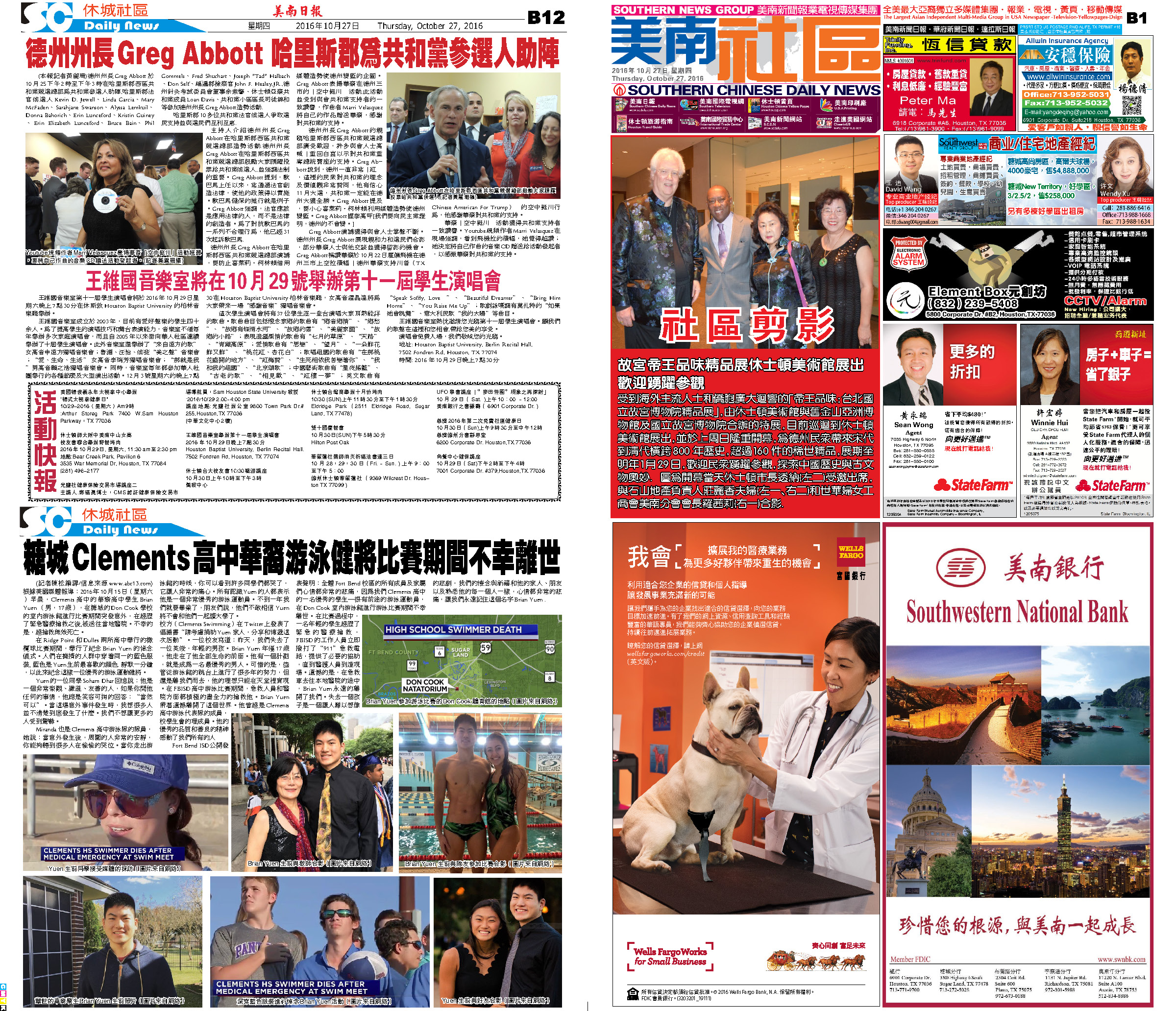C Section D Section